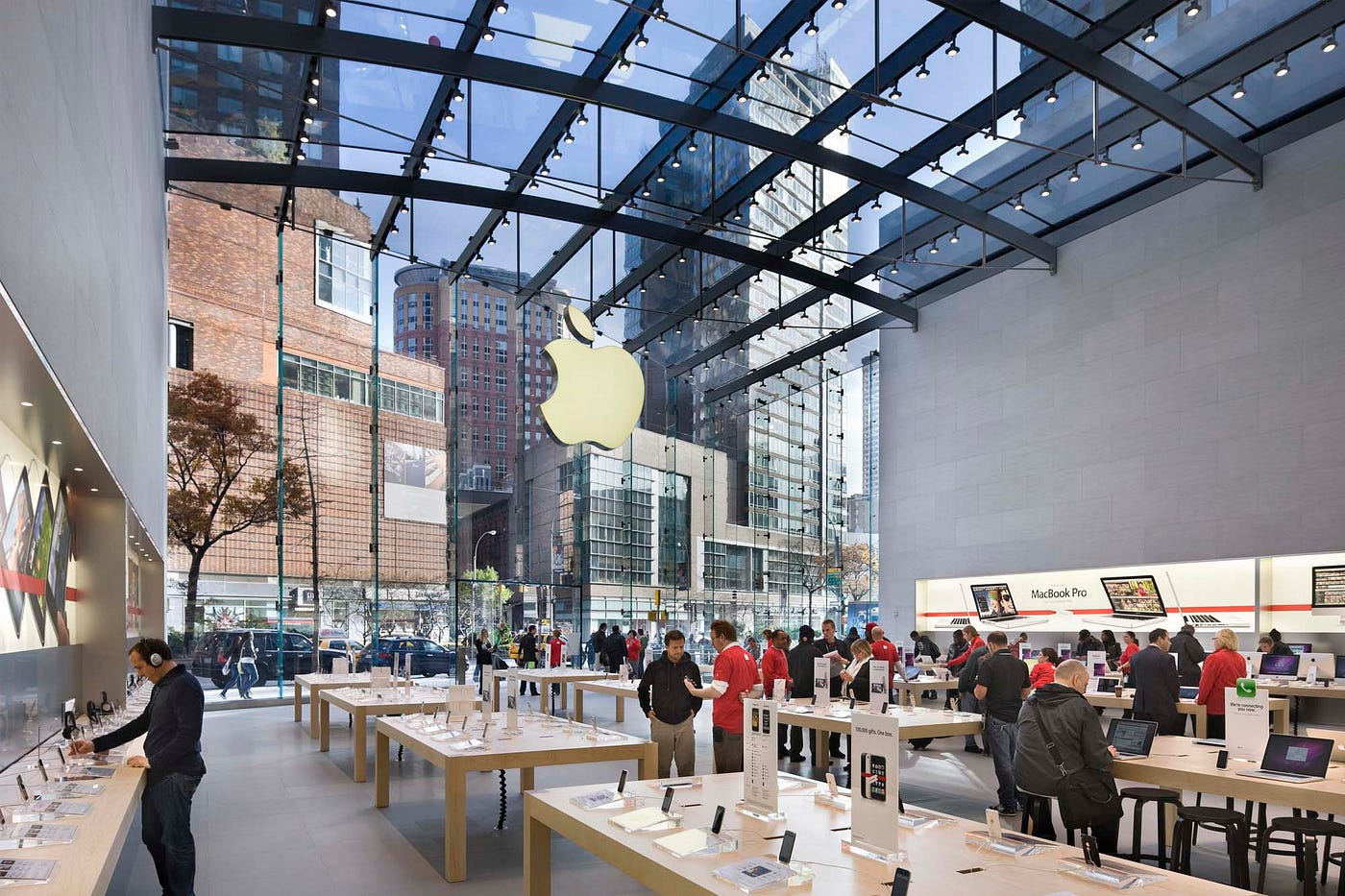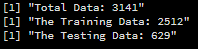# Introduction

The smartphone domain is an ever-changing as well as a challenging space to navigate. It is no surprise that there are more smartphone users in the world than desktop or laptop users. According to IDC, Android users have about 83.8% of the smartphone market compared to iOS which is about 16.2% in the year 2021. Mobile app analytics is a great way to understand the existing strategy to drive growth and retention of future users in this competitive market. This data set deals with the iOS App Store. The data contains various information about 7197 apps. However, this data set is from 2017. This project attempts to analyse this data so as to provide better insights and broaden the perspective of the reader with respect to Apple products. The project deals with 6 main questions:-

1. How do you visualize price distribution of paid apps ?
2. How does the price distribution get affected by category ?
3. What about Paid Apps Vs Free Apps ?
4. Are paid apps good enough ?
5. As the size of the app increases do they get pricier ?
6. How are the apps distributed category wise ? Can we split by paid category ?

These questions are a part of the tasks regarding this data set in the popular data science website Kaggle which also happens to be the source for this data set(Link:- click here). The programming language of choice for the analysis of given data is R. The reader may also perform this with any other programming language of their choice.This picture is taken from Architect Magazine. A glimpse of heaven on earth indeed

The Attributes for the data set are as follows:-

1. “id” : App ID

2. “track_name”: App Name

3. “size_bytes”: Size (in Bytes)

4. “currency”: Currency Type

5. “price”: Price amount

6. “rating_count_tot”: User Rating counts (for all version)

7. “rating_count_ver”: User Rating counts (for current version)

8. “user_rating” : Average User Rating value (for all version)

9. “user_rating_ver”: Average User Rating value (for current version)

11. “cont_rating”: Content Rating

12. “prime_genre”: Primary Genre

13. “sup_devices.num”: Number of supporting devices

14. “ipadSc_urls.num”: Number of screen shots showed for display

15. “lang.num”: Number of supported languages

16. “vpp_lic”: VPP Device Based Licensing Enabled

The data is actually consists of 7197 rows and 17 columns. But for convenience sake the first 6 rows are loaded. The initial data looks as follows:-

# Data Cleaning

Now before we begin analyzing the data set, there are a lot of changes I have made to the data in order to make it usable. The changes are as follows:-

1.) Eliminate the columns X and Currency. X is the index value which we can obtain by default every time we load any data set in R and Currency has only 1 value that being USD(United States Dollars).

2.) Conversion of size in Bytes to size in Mega Bytes and also renaming size_bytes to size_MB.

3.) Rearranging the KPIs as categorical string based data first and numerical data last.

4.) Omitting the Null Values( which to my surprise did not exist in the data set).

Now we can take a look at the cleansed data set and use it for further analysis:-

# Correlation Between All The Numerical Attributes

It is evident that the correlation between the attributes is very low. So the mathematical models based on this data will under perform.

# Data Separation

For convenience, the data is filtered using the filter function from dplyr package in the tidyverse package and put in a separate data frame. Thus, the entire cleaned is split into 2 data frames which are:-

1. Paid Apps Data Frame(PADF):-

2. Free Apps Data Frame(FADF):-

These will be useful as we shall be using these data frames to answer the questions above. Further for the last question, there are data frames that are created from the main data set using K-Means Clustering with respect to categories which are:-

GenreDF:- Prime Genre(prime_genre) Based Data Frame

ContentDF:- Content Rating(cont_rating) Based Data Frame

UserRatingDF:- Total User Rating(user_rating) Based Data Frame

1) How do you visualize price distribution of paid apps ?

Ans: According to me, the price distribution of paid apps can be best visualized as a vertical bar plot.

Clearly, there are a lot of free applications in this data set. Most of the applications cost less than $10. 2) How does the price distribution get affected by category? Ans: The Price Distribution gets affected by the categories as follows:- a.) Prime Genre Category:- An observation can be made that except for business related apps, mostly all the other applications have their prices below$10 max with a few exceptions. The categories that significantly maintain this trend are Books, Games and Entertainment.

b.) Content Rating Category:-

The 4+ and 12+ apps steal the spotlight for the applications with less than or equal to the $10 price mark. c.) User Rating Category:- Majorly 4 and 4.5 rated apps have the price mark of up to$10.

3) What about Paid apps Vs Free apps ?

Ans: Well, let’s start off by splitting the data into 2 cluster based data frames using K -Means clustering technique and plot the pie chart of the various user ratings. Here the KPI considered for measurement is user_rating of the application and that too the total user ratings not the one based upon individual version based ratings.

Paid Apps:-

The total data in the data frame(table) is 3141.

Free Apps:-

The total number of data in the data frame(table) is 4056.

The total number of data points in the Free Apps Data Frame is 4056 whereas that of the Paid Apps Data Frame is 3147. When it comes to the 4.5 rating(highest rating) category, free apps are 1465 compared to that of the price apps having 1198.

Now let us compare the percentage of free apps to that of the paid apps:-

The formula:- (No. of 4.5 rated apps/total number of apps)*100

 “Free apps 4.5 UR percentage: 36.1193”

 “Paid apps 4.5 UR percentage: 38.1407”

Now it is evident from the above percentage values that paid apps are having more apps with 4.5 user ratings. But I believe this truly does not give us the best results to understand which one is better. I think we need to take a look at which of the categories of apps are more likely to get a 4.5 star rating. So, I have prepared a mathematical model to model the data based on Data Classification techniques. The idea is to predict which app paid or free will be more likely to be awarded a 4.5 or above rating. The Model based upon the attributes having correlation greater than or equal to 0.05 is as follows( note that the price variable is 0 in free apps so it will not be added to the model):-

user_rating=β0+β1*size_MB+β2*rating_count_tot+β3*rating_count_ver+ β4 *vpp_lic

Three classification tests were performed(as per my knowledge) which are:
1.) K Nearest Neighbour
2.) Multinomial Logistic Regression
3.) Linear Discriminant Analysis

1. ) K Nearest Neighbours :

Free Apps:

Paid Apps:

2.) Multinomial Logistic Regression:

Free Apps:

Paid Apps:

3.) Linear Discriminant Analysis:

Free Apps:

Paid Apps:

Now truly the most miraculous thing happened! The accuracy of the test case for paid apps user rating prediction using LDA gives us an accuracy of 1 where as all of the others linger at a maximum accuracy of about 0.40. This is actually quite remarkable.

However this does not in anyway make this model to be any useful as the free apps prediction are less accurate. So, the model of our choice is going to be the K Nearest Neighbour since the accuracies of both the free and paid apps are more or less close to each other respectively. The result shows that the free apps are predicted by the model to more likely get a 4.5 rating than that of the paid apps. Although, the population of the free and the paid apps vary significantly. So how to find which one is correct.

Well the answer is simple. Take the percentages of these 2 app categories(free and paid) and compare them.

Hence, Paid Apps may be better than Free Apps.

4) Are paid apps good enough ?
Ans: The test above does not confirm that the free apps are not better than paid apps. It may not be the case every time. But yes with an impressive 1198 out of 3147, it can be said that it is good but may not be good enough.

5) As the size of the app increases do they get pricier ?
Ans: To find out about the relationship between price and size of an app, let us first take a look at it’s correlation.

The correlation of these two attributes are not very significant. To visualize the spread of the data, the scatter plot come to our aid.

Now if we watch the this plot we get to know that majority of the apps some of them even being priced abut 300 dollars lie inside the 1 GB mark. Now we can see that the apps with the biggest of the size is costing the customer about a minimum of 1 to a maximum of 30 dollars.

But,to better understand the price and size relationship, let us build a linear regression model to model the data mathematically. The idea here is to really see whether the size of an app estimates the price of it.

The equation: $price=\beta_0+\beta_1*size$

The data is split into training data and testing data:-

The initial price models are as follows:-

Now lets plot the model to get more insights.

We see that the data is not normal by observing the QQ-plot and the Shapiro-Wilks Normality Test. So I am going to apply the Box-Cox method to try and convert the distribution into normal. The Box- Cox Method has the following formula to convert the data into a Normal distribution.

We obtain Lambda to be as follows:-

Now after conversion the data is as follows:-Note that the data becomes lesser as the data gets transformed into normal data.

The new normalized model is as follows:-

The New Price Model Plot:-

The QQ-plot shows that the data has improved but not significantly. The Shapiro-Wilk Normality test still shows the data to be not normal.

The r-squared and the adjusted r-squared are close to 0.099 which indicates low significance of the 2 variables price and size respectively.

Now time to predict some data for cross reference:

The predictions are inaccurate across all the values. So at this point we cannot say that as the price increases the size of the app also increases.

6) How are the apps distributed category wise ? Can we split by paid category ?
Ans:
The categorization of the data is done using K-means Clustering center=1.

a.) Genre based Categorization:-

b.)Content based Categorization:-

c.) User Rating Based Categorization:-

Paid Apps based categorization:-

This is accomplished using a simple function where an extra column is added to the main cleaned data set and the price values are compared within a simple for loop to mark the respective row values as:-

0=’Free’
1=’Paid’

# Conclusion

This data is 4 years old. So, with new data the answers may change. Similar questions can be answered with an Android data set.

--

--